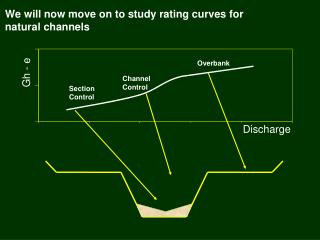# We will now move on to study rating curves for natural channels - PowerPoint PPT PresentationDownload PresentationWe will now move on to study rating curves for natural channels

We will now move on to study rating curves for natural channels
Download Presentation## We will now move on to study rating curves for natural channels

- - - - - - - - - - - - - - - - - - - - - - - - - - - E N D - - - - - - - - - - - - - - - - - - - - - - - - - - -
##### Presentation Transcript

1. We will now move on to study rating curves for natural channels Overbank Gh - e Channel Control Section Control Discharge

2. The relation between stage and discharge in a natural channel can also be assumed to be a power function

3. Here is the power function that is often used to relate discharge and head under section control Q = a(GH-e)b where: a = coefficient b = slope of the relation (b is almost always greater than 2)

4. Here is the equation that is often used to relate discharge and head under channel control Manning’s equation: Q = 1.486 A R 2/3 S 1/2 n Where: A = cross section area R = hydraulic radius (area/wetted perimeter) S = energy slope n = Manning’s “n” (roughness coefficient)

5. Manning equation can be reduced to a simple power function Q = 1.486 A R 2/3 S 1/2 n Can be reduced to: Q = C d1.67 = C (GH-e)1.67 Clickhere if you want to see how this is done.

6. Just to be sure we all know how to use exponents and how to compute basic hydraulic parameters we will go through a brief math primer • Weir equation: • Q = CBH 3/2 • where • C = 2.5, • B = Top width = 20 ft • H = Average depth of flow = 2 ft Q = 2.5 x 20 x 2 1.50 = 141 ft3/s

7. Math Primer (cont) • Manning equation: • Q = 1.486 AR 2/3 S 1/2 • n • where: • n = roughness coefficient = 0.05 • A = cross sectional area = 500 ft2 • R = Hydraulic Radius (area / wetted perimeter) = 4.5 ft • S = Energy slope (assume to be same as water surface slope = 0.002 ft/ft Q = 1.486 x 500 x 4.5 .667 x 0.0021/2 = 0.05 1812 ft3/s

8. Math Primer (cont). Compute area for gh = 10 ft 10 ft Overflow 5 ft Sand bar 0 ft 100 ft 0 ft 200 ft Area for main channel = (10 x 100) – ((5 x 100)/2) = 750 ft2 (5 x 100) = 500 ft2 Area for overflow = TOTAL AREA = 1250 ft2

9. Math Primer (cont.) Note: WP does not increase on main channel left bank above gh of 5 ft. There is no boundary friction there. Compute WP, and R for gh = 12 ft 12 ft Overflow Main Channel 500 ft2 1000 ft2 7 ft 2 ft 100 ft 0 ft 200 ft 10 + 100 + 5 = 115 ft WP for main channel = 100 + 5 = 105 ft WP for overflow = TOTAL WP = 220 ft R = A / WP = (1000 + 500) / 220 = 6.82 ft

10. Rating curve slopes can provide insights to control conditions Overbank Channel Control Gh - e Section Control 1 <2 1 >2

11. Here is how to measure rating curve slopes Measure down one unit then measure horizontal distance to rating using same unit of measure slope. Rating must be straight line and scales cannot be distorted! 1 <2 (usually 1.3 to 1.8) 1 >2

12. An example of a rating and controls PZF = 0.07 G.H. = 5.4 G.H. = 6.25 Click here to see video of control at high flow (1.2 MB file)

13. Artificial controls can also have compound shapes Artificial section control

14. Multiple offsets can be used to straighten different parts of ratings • Use of multiple offsets makes sense for many ratings • GRSAT making working with multiple offsets easy! Offset = 3 Offset = 1

15. You must smooth the transition between rating segments when multiple offsets are used! Click on the photo below to view an animation of changing controls

16. It is often necessary to extend ratings to stages above or below where measurements have been made ? ? ? ?

17. Ratings can be extended to low stages by: • Using arithmetic-scale graph and the PZF as a guide. • Using knowledge of the control • Using hydraulic equations Point of zero flow

18. Ratings can be extended to high stages by: • Plotting techniques • Use straight line, knowledge of control. • Slope-conveyance • Step-backwater model • Areal comparison of peak runoff rates • Flood routing

19. Here is a rule of thumb to keep in mind when extending ratings • Rule of Thumb -- Do NOT extend beyond twice the highest measured discharge (this may be a direct or indirect measurement)

20. You can be much more certain of how to extending the rating when the correct offset is used! Straight line extension 20 15 6

21. Rating extensions must consider channel geometry Overbank Gage height Channel Control

22. When to Draw a New Rating? • When measurements indicate a significant, consistent, and permanent departure from the current rating, especially on the upper end. • When the shape of the shift diagrams departs significantly from the shape of the rating.